# Series Reaction - Chemical Kinetics Chemistry Notes | EduRev

## Chemistry : Series Reaction - Chemical Kinetics Chemistry Notes | EduRev

The document Series Reaction - Chemical Kinetics Chemistry Notes | EduRev is a part of the Chemistry Course Physical Chemistry.
All you need of Chemistry at this link: Chemistry

Consecutive elementary reaction (Series reaction):

Consider the following series reaction scheme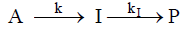In this, the reactant A decays to four intermediate I, and this intermediate undergoes subsequent decay resulting in the formation of product P. The above series is elementary first order reaction.
Then the rate law expression is :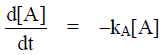…(1)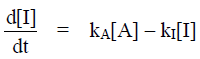…(2)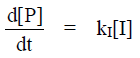…(3)

Let only the reactant A is present at t = 0 such that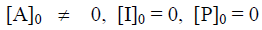then the rate law expression is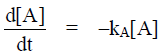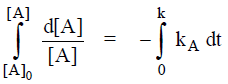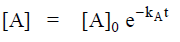…(4)
The expression for [A] is substituted into the rate law of I resulting in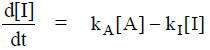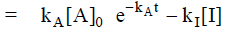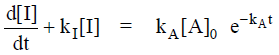This differential equation has a standard form and after setting

[I]0 = 0, the solution is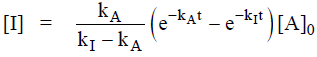The expression for [P] is

[A]0 = [A] + [I] + [P]

[P] = [A]0 – [A] – [I]
So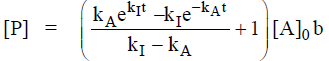Case I. Let                    kA >> kI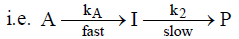then

kI – kA ≈ –kA
and e-kA≈ 0
∴[P] = [A]0 – [A] – [I]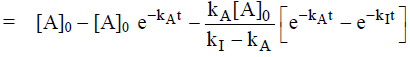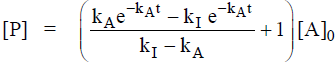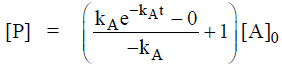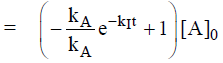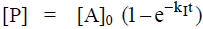The rate of formation of product can be determined by slowest step.

[A] = [A]0 e-kAt                                                          ............(1)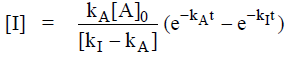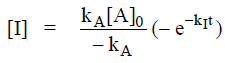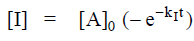...........(2)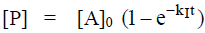............(3)

The graph representation for case I i.e. when kA >> kI.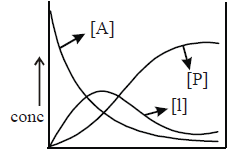Case II. kA >> kI

kI – k≈ kI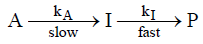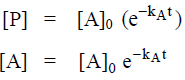The graph representation of case II i.e. when kA >> kI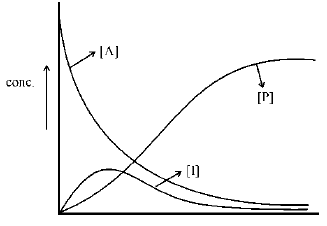The steady-state approximation assume that, after an initial induction period, an interval during which the concentration of intermediate ‘I’ rise from zero, and during the major part of the reaction, the rates of change of concentration of all reaction intermediate are negligibly small.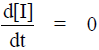Problem.  Consider the following reaction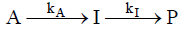assuming that only reactant A is present at t = 0, what is the expected time dependence of [P] using the steady state approximation ?
Sol.
The differential rate expression for this reaction are: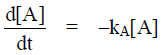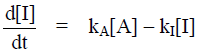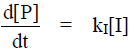Applying the steady state for I we get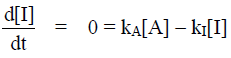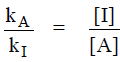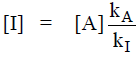and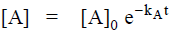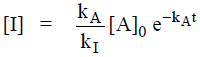then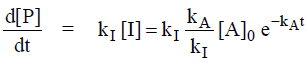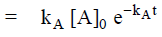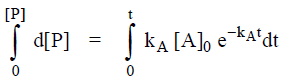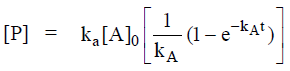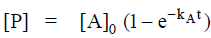This is expression for [P].

Problem. Using steady state approximation find the rate law for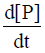for the following given equation.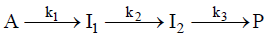Sol.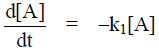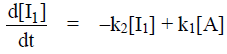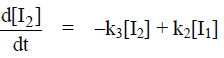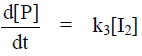I1 & I2 are intermediate & apply steady state approximation on intermediate, we get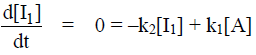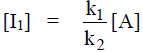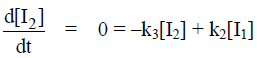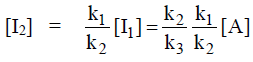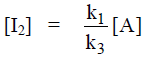and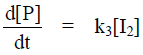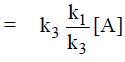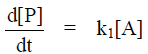Problem.  Using steady state approximation, derive the rate law for the decomposition of

N2O5. 2N2O5(g) → 4NO2(g) + O2(g)

On the basis of following mechanism.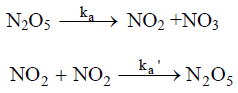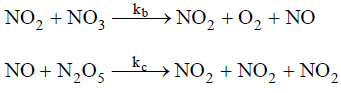Sol. The intermediate are NO & NO3.
The rate law are: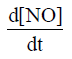= kb[NO2[[NO3] – kc [NO][N2O5] = 0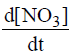= ka[N2O5] – ka’ [NO2][NO3] – kb[NO2][NO3] = 0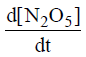= –ka[N2O5] + ka’[NO2][NO3] – kc[NO][N2O5]

and replacing the concentration of intermediate by using the equation above gives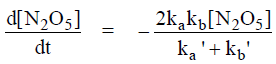Offer running on EduRev: Apply code STAYHOME200 to get INR 200 off on our premium plan EduRev Infinity!

## Physical Chemistry

77 videos|83 docs|32 tests

,

,

,

,

,

,

,

,

,

,

,

,

,

,

,

,

,

,

,

,

,

;